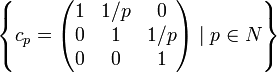# Zalesskii group

1 Partition the set of all prime numbers into three pairwise disjoint subsets$L,M,N$ where$2 \in N$, all three of the subsets being infinite.
2 Let$A$ be the set$\left \{ a_p = \begin{pmatrix} 1 & 1/p & 1/p^2 \\ 0 & 1 & 0 \\ 0 & 0 & 1 \\\end{pmatrix} \mid p \in L \right \}$
3 Let$B$ be the set$\left \{ b_p = \begin{pmatrix} 1 & 0 & 1/p^2 \\ 0 & 1 & 1/p \\ 0 & 0 & 1 \\\end{pmatrix} \mid p \in M \right \}$
4 Let$C$ be the set$\left \{ c_p = \begin{pmatrix} 1 & 1/p & 0 \\ 0 & 1 & 1/p \\ 0 & 0 & 1 \\\end{pmatrix} \mid p \in N \right \}$
5 The group$G$ we are interested in is the subgroup$G = \langle A \cup B \cup C \rangle$ inside$UT(3,\mathbb{Q})$, the group of$3 \times 3$ upper-triangular matrices with 1s on the diagonal and rational entries.